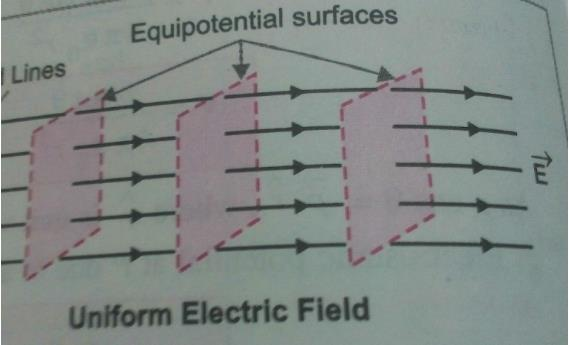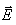Courses

# Test: Equipotential Surfaces

## 10 Questions MCQ Test Physics Class 12 | Test: Equipotential Surfaces

Description
This mock test of Test: Equipotential Surfaces for JEE helps you for every JEE entrance exam. This contains 10 Multiple Choice Questions for JEE Test: Equipotential Surfaces (mcq) to study with solutions a complete question bank. The solved questions answers in this Test: Equipotential Surfaces quiz give you a good mix of easy questions and tough questions. JEE students definitely take this Test: Equipotential Surfaces exercise for a better result in the exam. You can find other Test: Equipotential Surfaces extra questions, long questions & short questions for JEE on EduRev as well by searching above.
QUESTION: 1

Solution:

Definition based

QUESTION: 2

### Two equipotential surfaces have a potential of -10V and 90V respectively, what is the difference in potential between these surfaces?

Solution:

Potential = high potential - low potential
so our high potential is 90V and low potential is 10V,
potential = 90-(-10)=100V

QUESTION: 3

### Optical analogue of an equipotential surface is

Solution:

Definition based

QUESTION: 4

Electric field is steepest in the direction in which the potential

Solution:

In the direction of electric field the electric potential decreases. This is because electric potential is the work done against the direction of the electric field.

QUESTION: 5

Equipotential surfaces cannot

Solution:

The electric field lines are perpendicular to the equipotential surface. The field lines can not intersect each other because the electric force can not have two directions at a point.

QUESTION: 6

The shape of equipotential surface for an infinite line charge is:​

Solution:

The shape of equipotential surface for an infinite line charge is coaxial cylindrical because A curved surface on which potential is constant is equipotential curve . If we consider the line charge then the focus of the point should have the same potential hence it is a coaxial cylinder.

QUESTION: 7

When a positive charge is moved in an electrostatic field from a point at high potential to a low potential, its kinetic energy

Solution:

We can generalise it by assuming a positive charge moving away from another positive charge. now both of them are repelling each other with some force. so that positive charge will accelerate which results in the increase in K.E.

QUESTION: 8

Shape of equipotential surfaces for a uniform electric field along x-axis are

Solution:Shape of equipotential surfaces for a uniform electric field along x-axis are Planes normal to the field.

QUESTION: 9

The value of electric field vectoralong the surface of constant potential of 100 V

Solution:

As we know that electric field is in the reverse sense of the directional gradient of electric potential, so if V is constant, E should be zero. So, for E to be zero, either V has to be zero, or constant, or the 3 directional derivative components of V must cancel out each other in space. So we can not claim that V always has to be zero, if E is zero in a region, since E is a vector & V is a scalar quantity. But if V is zero, then surely E has to be zero; the reverse case is not always true.

QUESTION: 10

The amount of work done in moving a unit positive charge through distance of 10 cm on an equipotential surface is

Solution:

Definition based